);

# Quadrilaterals: GMAT / GRE Mathematics Tutorial

Contents

This tutorial is based on geometry concepts. We will study the types and properties of five quadrilaterals: Parallelogram, rectangle, square, rhombus and trapezium.

Basic definition

In Euclidean plane geometry, a quadrilateral is basically a four sided polygon, with the internal angles adding up to 360°.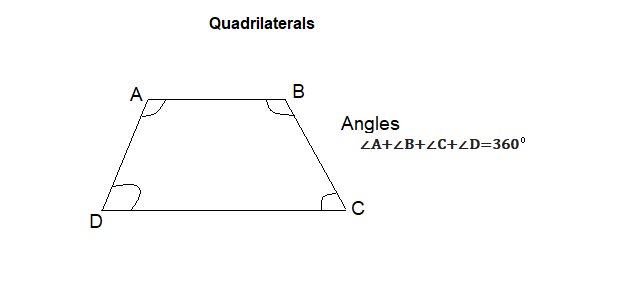Quadrilaterals are of five types based on their shapes. These are:

• Parallelogram
• Rectangle
• Square
• Rhombus
• Trapezium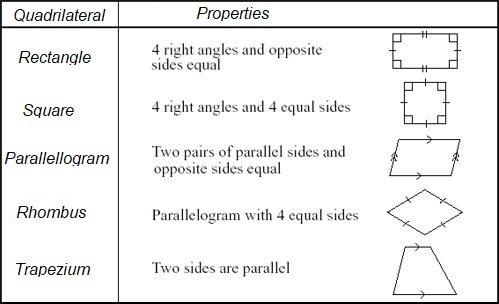We’ll study each of these quadrilaterals in detail.

## Parallelogram

A parallelogram is a quadrilateral with opposite sides parallel (as seen in the figure below).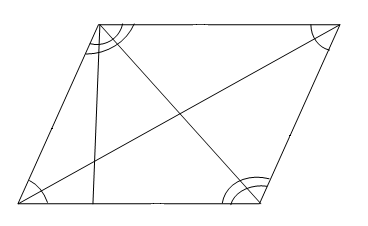### Properties of a parallelogram

• Opposite angles are equal.
• Opposite sides/facing sides are parallel and of equal length.
• Each diagonal divides the parallelogram into two equal triangles. The two diagonals bisect each other.
• If one of the angles of a parallelogram is a right angle then the parallelogram is a rectangle.

### Important formulas

If the length of a parallelogram is ‘l’, breadth is ‘b’ and height is ‘h’ then:

• Area = L * H
• Perimeter = 2(L+B)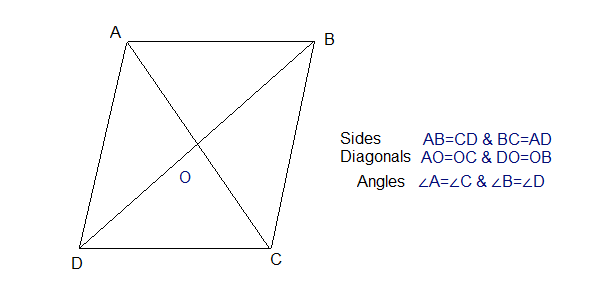## Rectangles

A rectangle is a quadrilateral with all angles at right angles (360°/4 = 90°). (As seen in the figure below)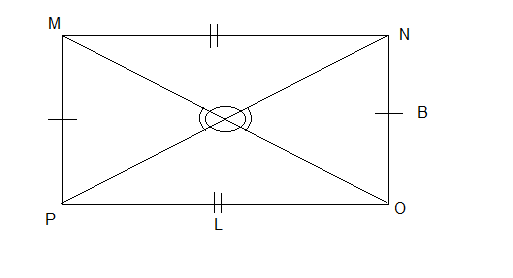Properties of a Rectangle

• Opposite sides are both parallel and equal to each other.
• All angles are 90°.
• The diagonals are equal and bisect each other (divide each other equally).
• Opposite angles formed at the point where diagonals meet are equal.
• A rectangle is a parallelogram with all the angles 90°.

Important formulas

• If L = length and B = breadth, then

Length of the diagonal = √ (L2 + B2)

• Area = L * B
• Perimeter = 2(L+B)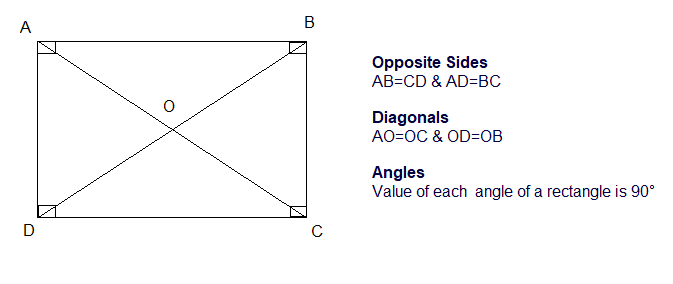## Squares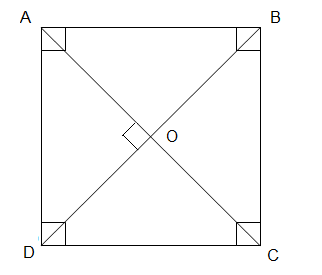A square is a quadrilateral in which all the sides are equal (as seen in the figure above).

Properties of a square

• All sides and angles are equal.
• Opposite sides are parallel to each other.
• The diagonals are equal and bisect each other at right angles.
• A square is a type of parallelogram in which all the angles and sides are equal.
• Also, a parallelogram becomes a square when the diagonals are equal and right bisectors of each other.

Important formulas for Squares

• If ‘L’ is the length of the side of a square then length of the diagonal = L √2.
• Area = L2.
• Perimeter = 4L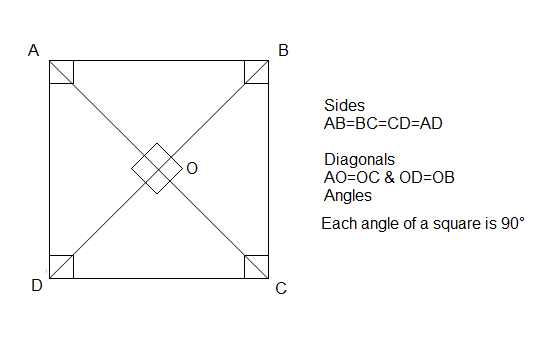## Rhombus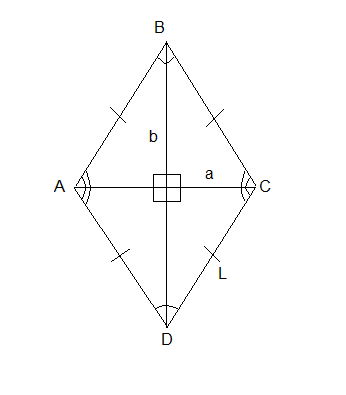A rhombus is a quadrilateral having equal sides and opposite sides are parallel to each other. (As seen in the figure above)

Properties of a Rhombus

• All sides are equal
• Opposite angles are equal
• The diagonals bisect each other at right angles
• Adjacent angles are supplementary (e.g. ∠A + ∠B = 180°)
• A rhombus is also a parallelogram that has diagonals that are perpendicular to each other
• A rhombus with right angles is a square

Important formulas for a Rhombus

If a and b are the lengths of the diagonals of a rhombus,

• Area = (a* b) / 2
• Perimeter = 4L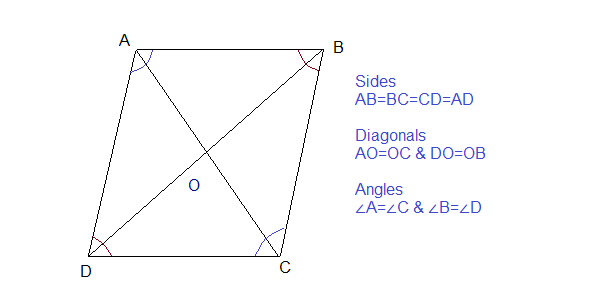## Trapezium

A trapezium or a trapezoid is a quadrilateral that has only one pair of parallel sides called bases and two lateral sides called legs.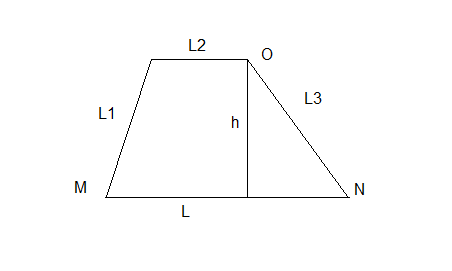Properties of a Trapezium

• Only the bases of the trapezium are parallel to each other (MN ⫽ OP).
• None of the sides, angles and diagonals are equal.

Important Formulas for a Trapezium

• Area = (1/2) h (L+L2)
• Perimeter = L + L1 + L2 + L3

## Summary of properties

Summarizing what we have learned so far for quick reference and recollection:

S.No.PropertyParallelogramRectangleRhombusSquare
1All sides are congruent
2Opposite sides are parallel and congruent
3All angles are congruent
4Opposite angles are congruent
5Diagonals are congruent
6Diagonals are perpendicular
7Diagonals bisect each other